# GMAT Math : Probability

## Example Questions

### Example Question #1 : Dsq: Calculating Probability

A fair coin is tossed a given number of times. What is the probability that three or more of the tosses will come up heads?

1) The number of times the coin is tossed is four.

2) The previous day, the coin was tossed ten times, and heads came up eight times.

BOTH statements TOGETHER are not sufficient to answer the question.

BOTH statements TOGETHER are sufficient to answer the question, but NEITHER statement ALONE is sufficient to answer the question.

Statement 1 ALONE is sufficient to answer the question, but Statement 2 ALONE is not sufficient to answer the question.

Statement 2 ALONE is sufficient to answer the question, but Statement 1 ALONE is not sufficient to answer the question.

EITHER statement ALONE is sufficient to answer the question.

Statement 1 ALONE is sufficient to answer the question, but Statement 2 ALONE is not sufficient to answer the question.

Explanation:

This is a binomial probability experiment.

The probability that a fair coin will come up heads in any single toss isIf the coin is tossed four times, then the probability that heads will appear three times is: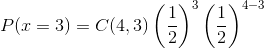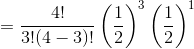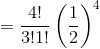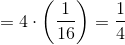The probability that heads will appear four times is: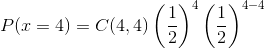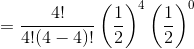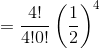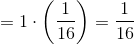So the probability that heads will appear at least three times is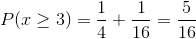The second statement is irrelevant; the result of a previous trial has no influence on that of the current trial. It has already been established in the problem that the coin is fair.

The answer is that Statement 1 alone is sufficient to answer the question, but not Statement 2.

### Example Question #61 : Data Sufficiency Questions

A monster is holding a brown paper bag filled with cookies. Inside the bag there are two types of cookies, chocolate chip and oatmeal raisin. If the monster pulls one cookie out at random, what is the probability that he pulls out a chocolate chip cookie.

1. There are a total of 24 cookies in the bag.

2. There are 8 more chocolate chip cookies in the bag than oatmeal raisin cookies.

BOTH statements TOGETHER are sufficient, but NEITHER statement ALONE is sufficient.

Statements (1) and (2) TOGETHER are NOT sufficient.

Statement (1) ALONE is sufficient, but statement (2) alone is not sufficient.

EACH statement ALONE is sufficient.

Statement (2) ALONE is sufficient, but statement (1) alone is not sufficient.

BOTH statements TOGETHER are sufficient, but NEITHER statement ALONE is sufficient.

Explanation:

We need both statements to get the answer.

Statement 1 alone is not enough. Having 24 total cookies does not tell us the proportions of each type of cookie. We could have 1 chocolate chip cookie and 23 oatmeal raisin, or vice versa.

Statement 2 alone is not sufficient either. Knowing that there are 8 more chocolate chip cookies than oatmeal raisin without knowing the total amount in the bag does not help. It could be 1 oatmeal raisin cookie and 9 chocolate chip, or it could be 100 oatmeal raisin cookies and 108 chocolate chip cookies (although that is a pretty big bag!)

Only by having both statements together can we find the right proportion. If we letbe the number of chocolate chip cookies, andbe the number of oatmeal raisin, we can set two equations and find the right ratio. We know that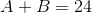from statement 1, andfrom statement 2.

Substitutinginto the first equation we get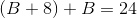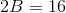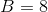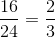or 66.67% chance that the monster pulls out a chocolate chip cookie.

### Example Question #1 : Dsq: Calculating Probability

Choose the answer that best describes sufficient data to solve the problem.

A student body is polled regarding their favorite campus food.  Of those who answered "Pizza" half were male and half were female.  Pizza received 24 votes from men.

I.  Pizza received the votes of 1 out of every 5 men and 3 out of every 10 women.

II. The student population was found to be 60% male and 40% female

How many students altogether were polled?

Either statement alone is sufficient to solve the problem.

Statement I is sufficient to solve the problem, but Statement II alone is not.

Neither statement is sufficient to solve the problem (additional information is needed).

Statement II is sufficient to solve the problem, but Statement I alone is not.

Both statements together are sufficient to solve the problem.

Statement I is sufficient to solve the problem, but Statement II alone is not.

Explanation:

Pizza received 24 votes from men. Since half of those who responded pizza were women, there must be 24 votes from women.

If 1 out of 5 men voted for pizza, this means we have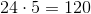men.  If 3 out of 10 women voted for pizza, we have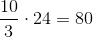women for a total of 200 people polled.

If our population is instead given as 60% male and 40% female, we still have 24 men and 24 women that voted for pizza, but while we can determine the ratio of people who voted, we cannot get a precise number.  For example, while 80 women and 120 men works, we could also have 160 women and 240 men as our total poll (with 1 out of 10 men and 3 out of 20 women voting pizza).

Thus the first condition is sufficient while the second is not.

### Example Question #1 : Probability

Find the probability.

Statement 1): Given that the lastflips of a coin were heads, the next coin flip is heads.

Statement 2): Given that the lastdays were sunny skies, the next day is sunny.

BOTH statements taken TOGETHER are sufficient to answer the question, but neither statement ALONE is sufficient.

Statement 1) ALONE is sufficient, but Statement 2) ALONE is not sufficient to answer the question.

Statement 2) ALONE is sufficient, but Statement 1) ALONE is not sufficient to answer the question.

BOTH statements TOGETHER are NOT sufficient, and additional data is needed to answer the question.

EACH statement ALONE is sufficient.

Statement 1) ALONE is sufficient, but Statement 2) ALONE is not sufficient to answer the question.

Explanation:

This question deals with conditional probability.

Statement 1) consists of independent events. The past trials will not affect the future trials of the coin.  The odds of flipping a coin and land on heads will be.

Statement 2) also consists of independent events. However, given that the previous 10 days were sunny does not necessarily indicate that the next day will guarantee that it will be a sunny day.  The probability is unknown because the statement does not give any information about the percentage of a sunny sky on any particular day.

### Example Question #64 : Word Problems

Jenkins has a box of 36 guitar picks. Each pick is one of three colors: blue, black, or orange.

I) There are 14 more orange picks than blue picks.

II) There are 12 black picks.

What are the odds of randomly selecting a black pick, followed by a blue pick, followed by an orange pick?

Statement II is sufficient to answer the question, but Statement I is not sufficient to answer the question.

Statement I is sufficient to answer the question, but Statement II is not sufficient to answer the question.

Both statements are needed to answer the question.

Either statement is sufficient to answer the question.

Both statements are needed to answer the question.

Explanation:

To find the probability of several events, we need to find the probability of each event separately. First, we need to know how many there are of each color pick.

Statement I relates the number of orange and blue picks.

Statement II tells us the number of black picks.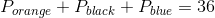From Statement I: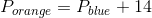From Statement II: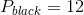Using Statement I, Statement II, and the given info, we can substitute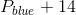from Statement I into Statement II for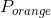and get the following: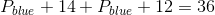We can then solve for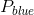: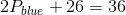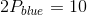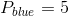Since we are told in Statement I that there are 14 more blue picks than orange picks, we can then find the number of orange picks: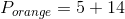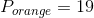So, to find the odds of multiple events, multiply the probability of each event together: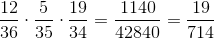### All GMAT Math Resources